Documentation

### This is machine translation

Mouseover text to see original. Click the button below to return to the English version of the page.

To view all translated materials including this page, select Country from the country navigator on the bottom of this page.

## Data Analysis on a Set of S-parameters for an RF Filter

This example shows how to perform statistical analysis on a set of S-parameter data files. First, read twelve S-parameter files representing twelve similar RF filters into the MATLAB workspace and plot them. Next, plot and analyze the passband response of these filters to ensure they meet statistical norms.

### Read in S-parameters from Filter Data Files

Use built-in RF Toolbox functions for reading a set of S-Parameter data files. For each filter, collect and plot the S21 raw values and S21 dB values. The names of the files are AWS_Filter_1.s2p through AWS_Filter_12.s2p and are located in the directory: MATLABROOT/toolbox/rf/rfdemos. These files represent 12 passband filters with similar specifications.

```numfiles = 12; numfreq = 801; % Number of frequency points per file for n = 1:numfiles filename = ['AWS_Filter_',num2str(n),'.s2p']; S = sparameters(filename); s21 = rfparam(S,2,1); s21_data(:,n) = s21; s21_db(:,n) = 20*log10(abs(s21)); end freq = S.Frequencies; % Frequency values are the same for all files figure plot(freq/1e9,s21_db) xlabel('Frequency (GHz)') ylabel('Filter Response (dB)') title('Transmission performance of 12 filters') axis on grid on ```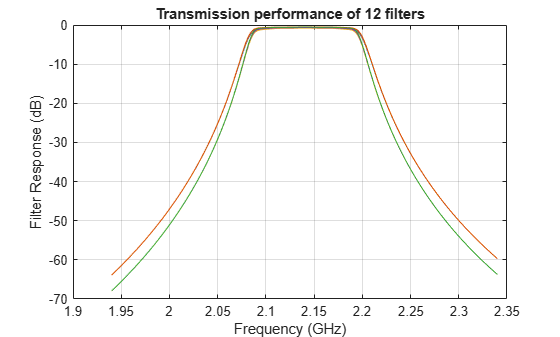### Filter Passband Visualization

In this section, find, store and plot the S21 data from just the AWS downlink band (2.11 to 2.17 GHz).

```idx = find((freq >= 2.11e9) & (freq <= 2.17e9)); freq_pass_ghz = freq(idx)/1e9; for n = 1:numfiles s21_pass_db(:,n) = s21_db(idx,n); s21_pass_data(:,n) = s21_data(idx,n); end plot(freq_pass_ghz,s21_pass_db) xlabel('Frequency (GHz)') ylabel('Filter Response (dB)') title('Passband variation of 12 filters') axis([min(freq_pass_ghz) max(freq_pass_ghz) -1 0]) grid on ```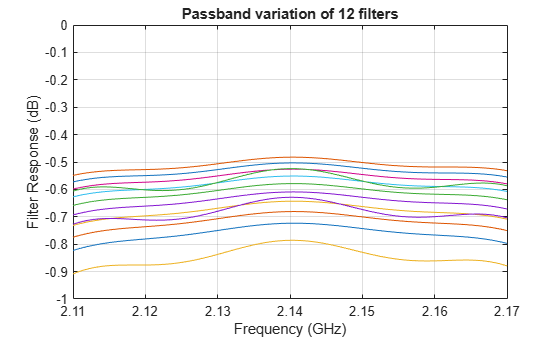### Basic Statistical Analysis of the S21 Data

Perform Statistical analysis on the magnitude and phase of all passband S21 data sets. This determines if the data follows a normal distribution and if there is outlier data.

```% Calculate the mean and standard deviation of the magnitude of the entire % passband S21 data set. mean_abs_S21 = mean(abs(s21_pass_data(:))) std_abs_S21 = std(abs(s21_pass_data(:))) % Calculate the mean and standard deviation of the passband magnitude % response at each frequency point. This determines if the data follows a % normal distribution. mean_abs_S21_freq = mean(abs(s21_pass_data),2); std_abs_S21_freq = std(abs(s21_pass_data),0,2); ```
```mean_abs_S21 = 0.9289 std_abs_S21 = 0.0104 ```

Plot all the raw passband magnitude data as a function of frequency, as well as the upper and lower limits defined by the basic statistical analysis.

```hold on plot(freq_pass_ghz,mean_abs_S21_freq) plot(freq_pass_ghz,mean_abs_S21_freq + 2*std_abs_S21_freq,'r') plot(freq_pass_ghz,mean_abs_S21_freq - 2*std_abs_S21_freq,'k') legend('Mean','Mean + 2*STD','Mean - 2*STD') plot(freq_pass_ghz,abs(s21_pass_data),'c','HandleVisibility','off') grid on axis([min(freq_pass_ghz) max(freq_pass_ghz) 0.9 1]); ylabel('Magnitude S21') xlabel('Frequency (GHz)') title('S21 (Magnitude) - Statistical Analysis') hold off ```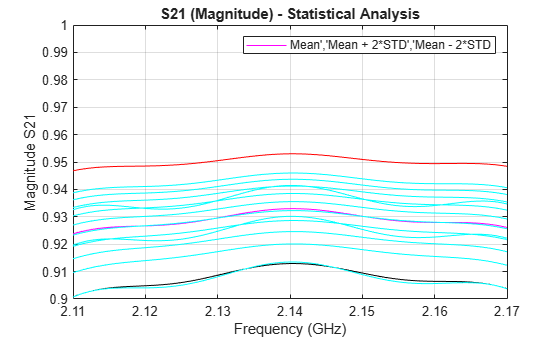Plot a histogram for the passband magnitude data. This determines if the upper and lower limits of the data follow a normal distribution.

```histfit(abs(s21_pass_data(:))) grid on axis([0.8 1 0 100]) xlabel('Magnitude S21') ylabel('Distribution') title('Compare filter passband response vs. a normal distribution') ```Calculate the phase response of the passband S21 data, then the per-frequency mean and standard deviation of the phase response. All the passband S21 phase data is then collected into a single vector for later analysis.

```pha_s21 = angle(s21_pass_data)*180/pi; mean_pha_S21 = mean(pha_s21,2); std_pha_S21 = std(pha_s21,0,2); all_pha_data = reshape(pha_s21.',numel(pha_s21),1); % Plot all the raw passband phase data as a function of frequency, as well % as the upper and lower limits defined by the basic statistical analysis. hold on plot(freq_pass_ghz,mean_pha_S21) plot(freq_pass_ghz,mean_pha_S21 + 2*std_pha_S21,'r') plot(freq_pass_ghz,mean_pha_S21 - 2*std_pha_S21,'k') legend('Mean','Mean + 2*STD','Mean - 2*STD') plot(freq_pass_ghz,pha_s21,'c','HandleVisibility','off') grid on axis([min(freq_pass_ghz) max(freq_pass_ghz) -180 180]) ylabel('Phase S21') xlabel('Frequency (GHz)') title('S21 (Phase) - Statistical Analysis') hold off ```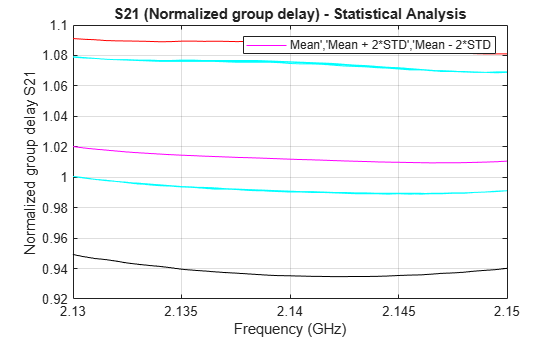Plot a histogram for the passband phase data. This determines if the upper and lower limits of the data follow a uniform distribution.

```histogram(all_pha_data,35) grid on axis([-180 180 0 100]) xlabel('Phase S21 (degrees)') ylabel('Distribution') title('Histogram of the filter phase response') ```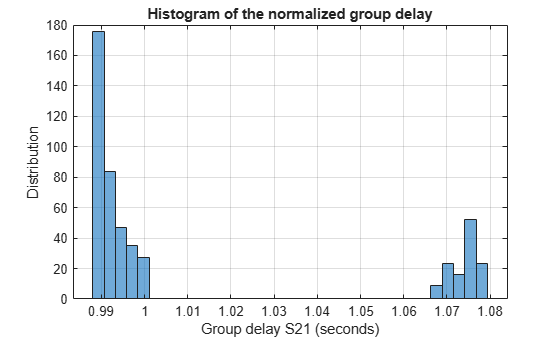### ANOVA Analysis of the S21 Data

```% Perform ANOVA analysis on both the magnitude and phase passband data. anova1(abs(s21_pass_data).',freq_pass_ghz); ylabel('Magnitude S21') xlabel('Frequency (GHz)') ax1 = gca; ax1.XTick = 0.5:10:120.5; ax1.XTickLabel = {2.11,'',2.12,'',2.13,'',2.14,'',2.15,'',2.16,'',2.17}; title('Analysis of variance (ANOVA) of passband loss') grid on ``````anova1(pha_s21.',freq_pass_ghz); ylabel('Phase S21 (degrees)') xlabel('Frequency (GHz)') ax2 = gca; ax2.XTick = 0.5:10:120.5; ax2.XTickLabel = {2.11,'',2.12,'',2.13,'',2.14,'',2.15,'',2.16,'',2.17}; title('Analysis of variance (ANOVA) of passband phase response') grid on ```### Fit the Phase Data to 1st-Order Polynomial

```% Perform a curve fit of the S21 phase data using a linear regression % model. phase_s21_fit = fit(repmat(freq_pass_ghz,numfiles,1),all_pha_data,'poly1') ```
```phase_s21_fit = Linear model Poly1: phase_s21_fit(x) = p1*x + p2 Coefficients (with 95% confidence bounds): p1 = -310.8 (-500.9, -120.6) p2 = 665.3 (258.3, 1072) ```